# 3D Simulation of Axial field Permanent Magnet coupling system using EMS and SOLIDWORKS Motion Coupling

HOME / Applications / 3D Simulation of Axial field Permanent Magnet coupling system using EMS and SOLIDWORKS Motion Coupling

### Applications

Used Tools:## Introduction

Permanent Magnet couplings (or couplers) are used to transmit torque from a primary driver to a secondary follower without any mechanical contact.  Many industrial applications are based on such transmission system that doesn`t need any external electrical circuit for motors and propellers. They are especially used for high security applications and in sealed equipment for transmissions through walls.

A mechanical configuration for such type of coupling can be damaged because of twisting when the applied torque is more than the rated quantity. Therefore, the absence of the direct mechanical contact overcome this problem by reducing the generated vibrations and mechanical friction losses. This can avoid the maintenance and repair aspects in the mechanical conventional coupling and then increases the lifetime of the system. This synchronous coupling type presents a maximum transmissible torque which gives an intrinsic protection against overloads.

Magnetic coupling could have two different topologies according to the flux directions: Axial or radial (face-to-face and Co-axial). They both consists of two rotors equipped by circular array of Permanent Magnets alternately magnetized along  direction and presents the same pairs pole number. Figure 2 shows the two different Permanent Magnet (PM) coupling designs.

In particular, the axial flux permanent magnet (PM) coupling topology has the advantage of compact structure and can achieve higher torque transmission. It is investigated in this study and it consists of two opposed Soft-Iron discs equally equipped with axially magnetized Permanent Magnets. The PM arrangement forms a north-south alternating polarization. Both rotors are separated by a well-adjusted air gap length which defines the torque control factor.

The torque characteristic of the magnetic coupler could be investigated and geometrically optimized using the FEM 3D electromagnetic field analysis by EMS. It is strongly related to the magnetic performance of : PM, number of poles, air gap distance and to the magnetic circuits. The Figure 3 shows the detailed geometry of the studied axial flux PM coupling device.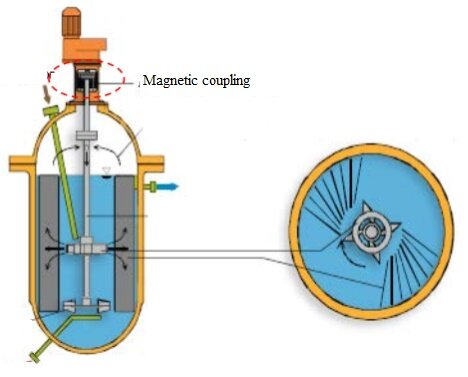Figure 1 - Magnetic coupling drives a turbine shaft in a chemical reactor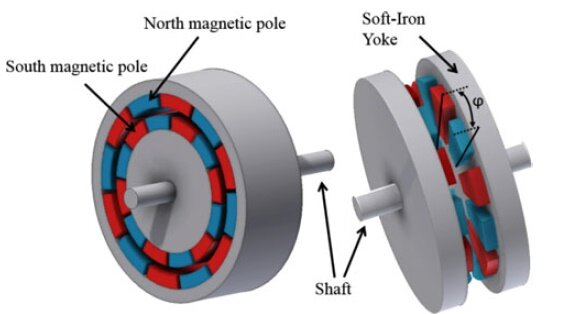Figure 2 - Topologies of the Radial flux and Axial flux magnetic coupling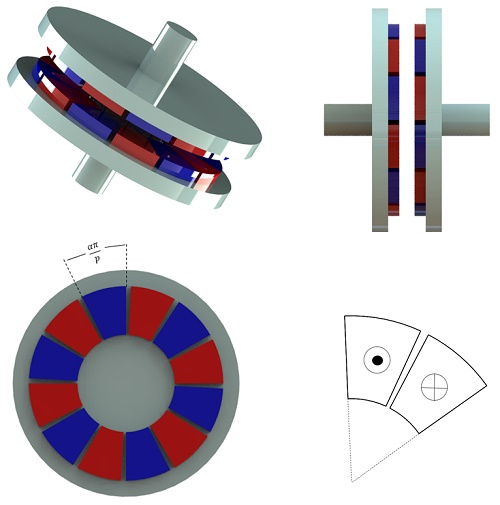Figure 3 - 3D design of the Axial flux magnetic coupling and schematic illustration of the alternative magnetization of PM poles.

## Problem description

The magnetostatic module is used to predict the field distribution and torque behavior versus geometrical criteria using the parametric study of EMS:  It allows to predict the effect of some of  those criteria on the magnetic performance of the coupling through the run of different scenarios in a single study by parametrizing:

1. The mechanical displacement angle (Load angle) between the two rotors for a fixed air gap
2. The air gap value between the parallel rotors for a fixed load angle.

The main geometrical dimensions are detailed below in Table 1:

Table 1 - Geometrical dimensions 
 Component Dimensions (mm) Outer radius 60 Inner radius 30 Outer yoke radius 70 Yoke thickness 10 PM thickness 7 Air gap thickness 9.5 Number of pole pairs 6 Magnet opening to pole-pitch ratio 0.9

The magnets are axially magnetized and have a relative permeability equal to 1. The ferromagnetic yokes have been chosen with a thickness of 10mm to avoid magnetic saturation and they are made of Soft iron material characterized by the non-linear BH curve figured below.
The table 2 defines the corresponding material properties mentioned above.

Table 2 - Material properties
 Material Magnetic permeability Electrical conductivityRemanence Coercivity Soft Iron-yoke BH curve 10 - PM-NdFeB 1 0 1.25 T 994718.4 A/m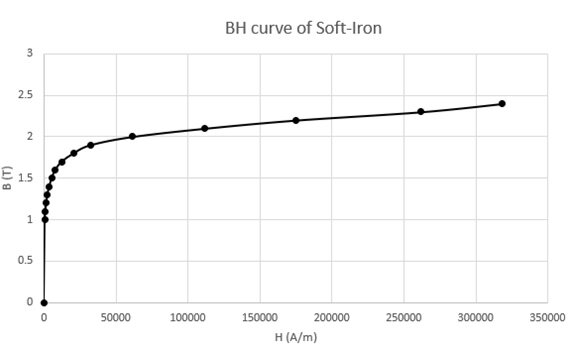Figure 4 - BH curve of Soft-Iron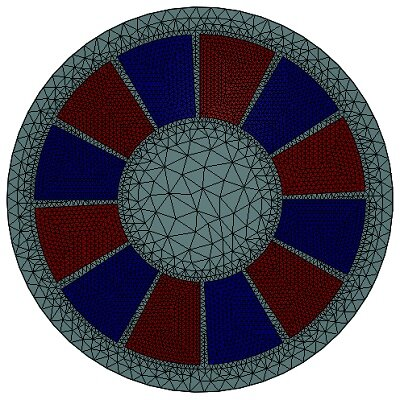Figure 5 - Meshed model

### Parametric study

Case 1:  By varying the mechanical displacement angle  between the similarly magnetized poles of the rotors: the solver computes the electromagnetic and static torque at each scenario for a fixed air gap value of 9.5 mm. A maximum static torque is attained for a load angle of 15°.

Figure6-a) and 6-b) show the axial magnetic flux density when the angle shift between both sides of coupler is respectively 0° and 15°. At 0° : the similarly polarized poles are totally aligned: the magnetic reluctance is minimum which lead to a minimum torque, while at 15° ; the magnetic reluctance is at its peak value. Therefore, the magnetic torque reaches its maximum at this position.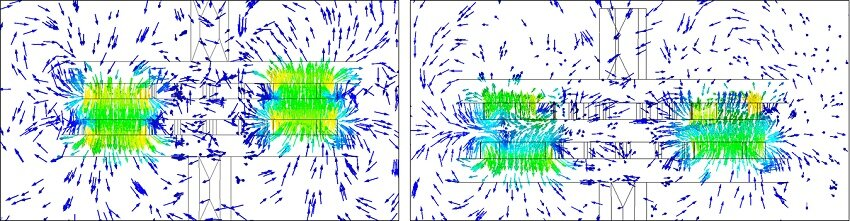Figure 6 -  Vector plot of Magnetic Flux between similarly magnetized poles forand.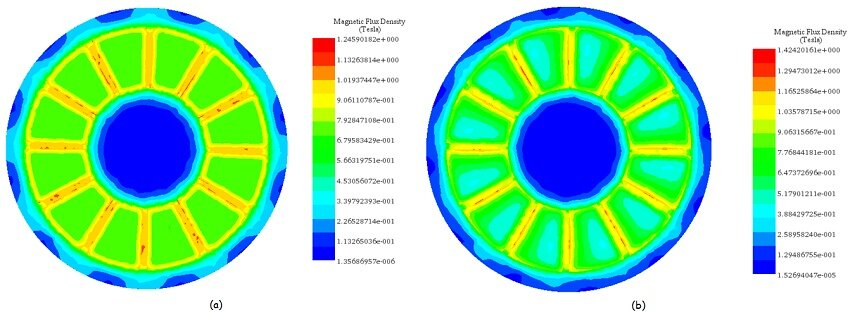Figure 7 -  Fringe plot of Magnetic Flux density at a single rotor for a)-and b)-.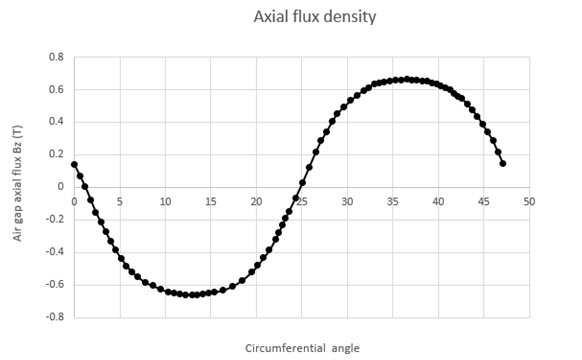Figure 8 - Axial flux density at mean radius of middle air gap versus circumferential angle.

The next figure confirms the good agreement between the experimental and FEM simulation results of EMS for the computed static torque versus angular displacement compared to the analytical calculations made inside the Ref . For an angular load bigger than 15°, the torque decreases until the next alignment with the next magnetized pole.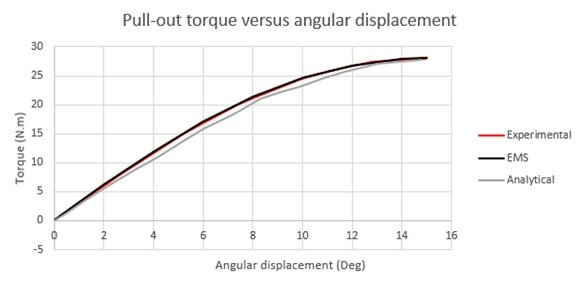Figure 9 -  Torque (Tz) versus angular displacement at air gap g=9.5mm

The flux density distribution along Z-axis is figured across the middle air gap at the center of the pole and compared to the Experimental and analytical results.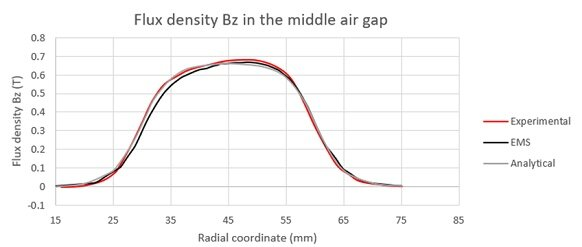Figure 10 - Axial flux density (Bz) versus radial coordinate at the center of pole at air gap g=9.5mm

Case 2:  For the second parametrization study, for a fixed mechanical load angle of 15°, the variation of the air gap distance revealed the next results: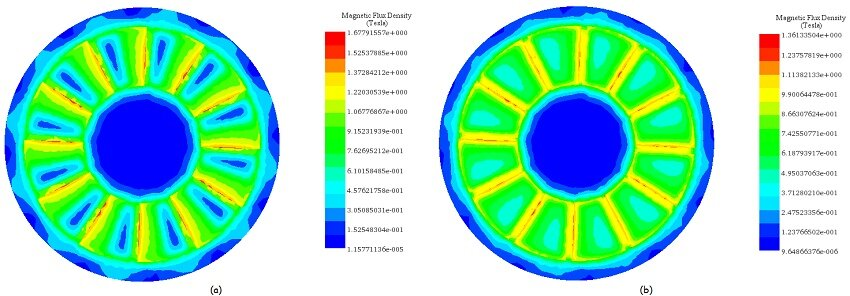Figure 11 - Fringe plot of Magnetic Flux density at a single rotor for air gap a)-and b)-at.

The pull-out torque of the studied magnetic coupling decreases quickly as the distance between the magnets increases. The maximum torque is almost divided by two when the air gap passes from 2mm to 7 mm, which is significant in our case.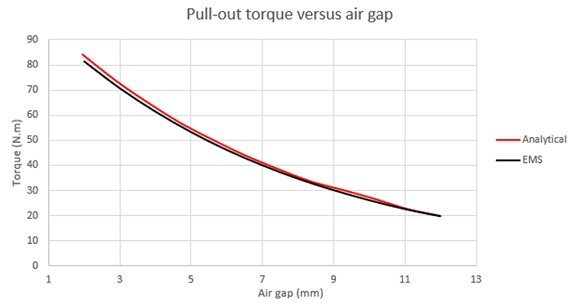Figure 12 - Pull-out torque versus air gap 

### Motion study

Case 1: For a fixed air gap of 9.5 mm and a constant angular speed of 500 rpm (3000 Deg/s) applied to the mover rotor and with fixing the second rotor;  the Motion study of SOLIDWORKS coupled to the magnetostatic study of EMS allows to compute the generated torque and angular displacement of the driver rotor versus time.

For a simulation time of 64 ms: The torque (Tz) is oscillating versus time between its maximum and minimum attained value according to the position of the polarized poles between each other.Figure 13 -  a)- Angular speed and displacement versus time b)-Torques results versus time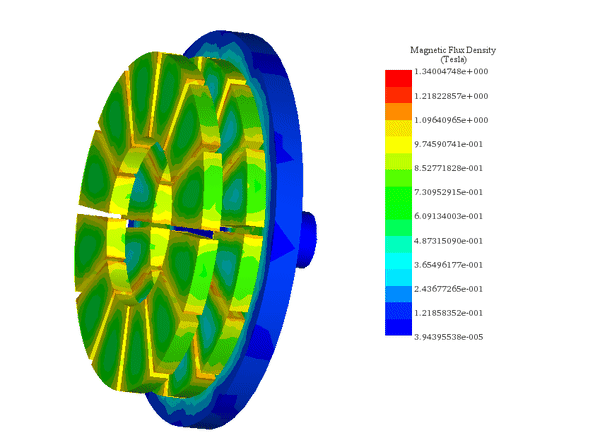Figure 14 - Animation of Magnetic flux variation versus time

Case 2: For a fixed air gap of 9.5 mm and a constant angular speed of 500 rpm applied to the mover rotor and with keeping the load angle ofbetween the similarly polarized poles:  the Motion study of SOLIDWORKS coupled to the magnetostatic study of EMS allows to compute the generated torque and speed of the follower rotor versus time.

Since it is a synchronous machine, if one side is rotating, the other rotates at the same speed and torque due to the fixed mechanical constraints that keeps the constant angular shift between them.

The first figure is showing the angular velocity and displacement at each rotor versus time and the second shows the dynamic torque results which have an averaging value around 27 N.m.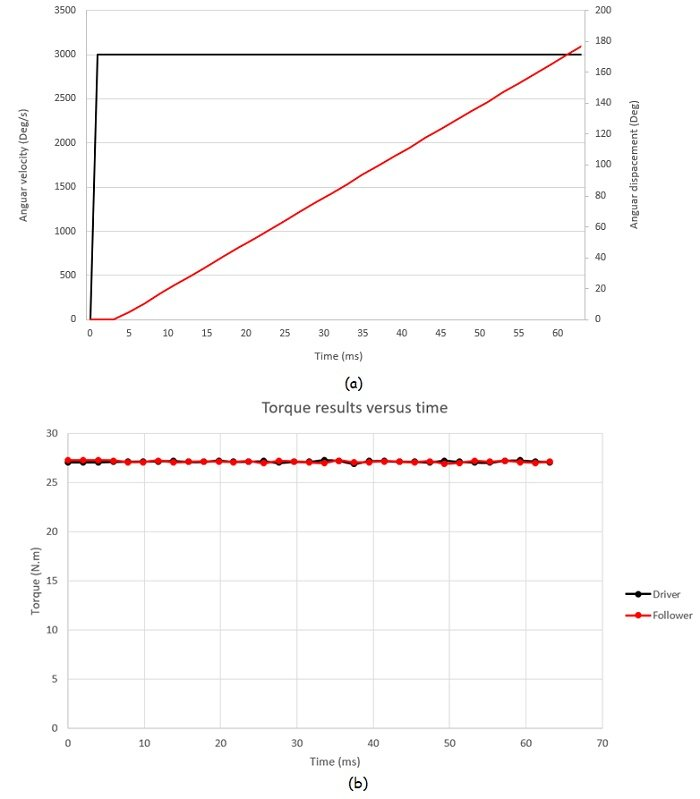Figure 15 -  a)- Angular speed and displacement versus time b)-Torques results versus time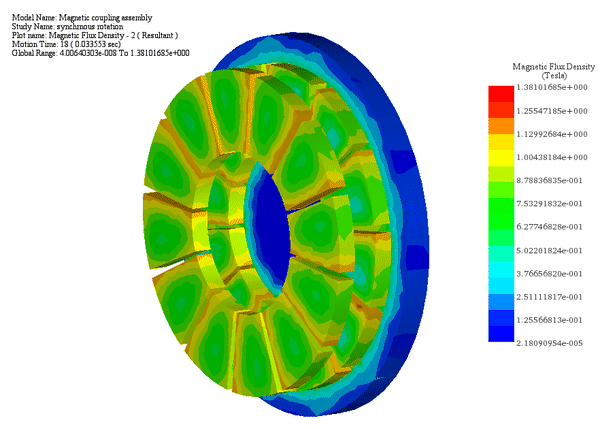Figure 16 - Animation of Magnetic flux variation versus time steps

## Conclusion

The use of permanent magnets in magnetic couplers is very interesting for applications requiring isolation between two different atmospheres without the need of mechanical contact between rotating parts. An accurate knowledge of the magnetic field distributions for different geometrical configurations is necessary for predicting the torque and speed behavior of such mechanical equipment.

The use of parametric study of EMS and motion analysis of SOLIDWORKS has shown a high capability of FEM tool to mechanically and electromagnetically analyze such complex systems under several conditions. The main advantage of axial-type magnetic coupling is the significant effect of magnetic field on transmitting torque between the rotating discs.

## References

 Dolisy, Bastien, et al. "A new analytical torque formula for axial field permanent magnets coupling." IEEE Transactions on Energy Conversion 30.3 (2015): 892-899.
 Dolisy, Bastien, et al. "Three-dimensional analytical model for an axial-field magnetic coupling." (2014).
 Lubin, Thierry, Smail Mezani, and Abderrezak Rezzoug. "Experimental and theoretical analyses of axial magnetic coupling under steady-state and transient operations." IEEE Transactions on Industrial Electronics 61.8 (2013): 4356-4365.